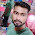### Program 350 : Frequency of all numbers in array

Program 350 : Frequency of all numbers in array

```#include<stdio.h>
#include<stdlib.h>
main()
{
int i,j,size,max;
printf("Enter size of array\n");
scanf("%d",&size);
int *a=(int*)malloc( size * sizeof(int) );
printf("Enter Elements\n");
for(i=0;i<size;i++)
{
scanf("%d",&a[i]);
}
max=a;
//Getting maximum number as we are going to check all numbers frequency from 0 till the max number of given elements
//to get numbers repeated odd times
for(i=0;i<size;i++)
{
if(max<a[i])
max=a[i];
}
max+=1;
//Temporary array to store frequency of numbers
int b[max];
//Resizing array to max number size
a=(int*) realloc(a,max*sizeof(int));
for(i=0;i<=max;i++)
{
b[i]=0;
if(i>=size)
a[i]=0;
}
//Storing frequency of all elements
for(i=0;i<=max;i++)
{
if(a[i]!=0)
b[a[i]]=b[a[i]]+1;
}
printf("Numbers repeated odd numbers of times\n");
for(i=0;i<=max;i++)
{
if(b[i]!=0)
printf("%d repeated %d times\n",i,b[i]);
}

}
```
Explanation:
//Coming Soon

Output:

#### 1 comment:

1.factorial hundred In the last few days, the “factorial of 100” is one of the top subjects and a lot of maths geeks compute it using voice assistants such as Alexa, Shiri, etc.

factorial hundred In the last few days, the “factorial of 100” is one of the top subjects and a lot of maths geeks compute it using voice assistants such as Alexa, Shiri, etc.

factorial hundred In the last few days, the “factorial of 100” is one of the top subjects and a lot of maths geeks compute it using voice assistants such as Alexa, Shiri, etc.

 Donate Buy me a coffee \$2.00 USD Buy me a burger \$5.00 USD Buy me a pizza \$10.00 USDCompiler Used by me C-Free 5.0(Recommended) Other Compilers Code Blocks(Recommended) Online Compilers Ideone(Remember to give input before executing online where ever necessary)